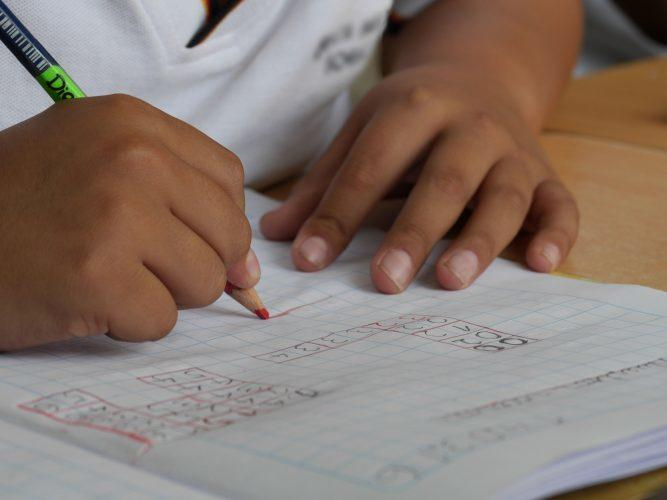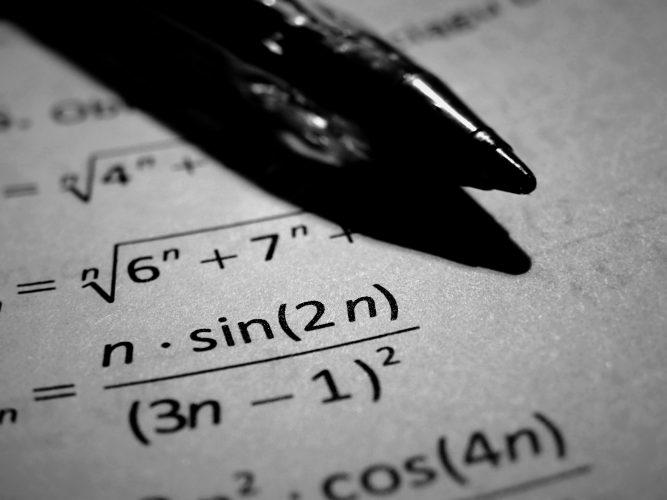Share

Maths Help with Equations, Variation and Sign Tables

By Jon, published on 31/03/2018 > > > How to Solve Maths Problems
• Advice For Solving Maths Problems
• How Do You Solve a Maths Equation?
• How to Solve Functions
• Unsolved Maths Problems

Maths can cause problems for children and adults alike. Do you panic when you have to solve a quadratic equation or have to think about graphing? It turns out that even physicists have worries when it comes to maths and algebraic problems!

How good are you when it comes to solving equations? Do you know your exponent from your elbow or are you in pieces just thinking about fractions?

A lot of people bemoan maths in schools, at A-level and GCSE maths revision, saying they’ll never use it in real life. However, the inverse is true. While you mightn’t use a particular theorem, your brain will often put them to use by substituting an approach into a real-life situation without you even realising.

Furthermore, adopting a rational and mathematical approach will allow you to not only solve all manner of problem and polynomial equation but also improve your memory.After learning to do sums, it’s time to get to grips with problems. (Source: pixabay.com)

These problems arise all the way through school. You have to know, if you want to get better at maths, how to simplify your problems and solve the equation, be it a linear equation or a quadratic formula.

Whatever the problem (or equation), the approach is always algebraically the same. When you solve equations, you usually have to solve for x. This means you’re given a formula with certain expressions, sometimes a coefficient, and your job is to rearrange it so that the unknown number (usually represented by x) becomes a known quantity.

Sometimes these problems are presented without the formula in the form of word problems whereby you have to create and solve equations, rather than just being given algebraic equations.

If you have gaps in your maths knowledge or you just want to improve, you have to master these essential maths skills.

Don’t forget that maths is a core subject and the skills you learn from it will accompany you throughout your academic and professional life!

Advice For Solving Maths Problems

Learning the necessary approaches to solve maths problems is at the heart of maths teaching. In fact, that’s why we deal with problems all the way through our maths lessons.

Whether it’s geometry or algebra, most of the exercises you do during your maths revision classes are also on the exams you take. Whatever your level, the approach to solving a maths problem is the same.

You have to imagine the problem like an investigation. You have the clues in the question and you have to carry out an inquiry and take the necessary steps to find the solution. If you want to succeed in maths, you have to know these concepts.

Whether in maths class or in a private tutorial, you’ll learn concepts that are essential to the discipline. Maths exercises are simply applying these concepts. Thus, revision sheets and studying the exercises done in class are essential to getting ahead in maths.If you want to solve maths problems, you have to pay attention in class! (Source: pixabay.com)

Once you’ve mastered these essential concepts for solving problems, you have to:

• take care of your work environment, keeping a calculator at hand

• take time to read the problem several times before starting to tackle it

• recognise the clues that you already have

• test hypotheses on a scrap piece of paper

• check your results several times

Being able to solve problems is essential if you want to progress in maths. Nevertheless, you may still have difficulties. In this case, you need to stay motivated and persevere.

A math tutor, through their private tutorials, can help a student to gain confidence in themselves and develop the essential maths skills.

If the problems are becoming a problem, the tutor can also provide regular training and adapt their teaching methods in order to help the maths student progress more quickly.

How Do You Solve a Maths Equation?

Before solving an equation, you need to understand what mathematicians mean when they say this.

An equation means that both sides are equal and there’s a variable, normally called x, which we need to find out.

We usually learn about addition, subtraction, division, multiplication, and mental arithmetic in primary school. However, it’s not until we’re older when we start to see equations popping up in this form:

7x + 5 = 3x – 15

This is when we first meet the x. and we start having to solve these equations which still involve adding, subtracting, dividing, and multiplying. As we move on through school, we start seeing more and more of them.

These equations get more complex in line with the level of maths taught as you get to the end of compulsory education.Equations are an integral part of secondary school maths. (Source: stock.tookapic.com)

Throughout your time in school and for those that decide to study maths or a scientific subject, these equations never disappear. So you’ll have to learn to solve them sooner or later!

The simplest equations (linear or first degree equations) are those that we first learn to solve and these are the steps you have to take to do it:

• Isolate the unknown (this means putting it on its own on one side of the equal sign)

• Regroup the terms

• Divide, multiply, add, and subtract (as per the equation)

• Write the solution

So, for example, the equation 3x – 5 = –x + 2 is solved as follows:

• 3x + x = 5 + 2
• 4x = 7
• x =  7/4

Therefore x = 1.75

Remember that when you solve equations, whatever you do to one side, you have to do to another, else you’ll end up with inequalities.

You should also remember that in order to find a solution, adding is the opposite of subtracting, dividing is the opposite of multiplying, taking the square root is the opposite of squaring, and factoring is the opposite of expanding.

Once you’ve worked how to solve linear equations, you can then move onto zero-product or second degree equations like: (ax + b) (cx+d) = 0.

The key rule that your maths teacher will tell you is that the product of these factors is zero only if one of the factors is zero. That means you need to solve as many equations as there are factors in your problem.

However, there are other types of quotient equations which can seem really complicated to students. Quotients are presented as follows: f(x) / g(x) = 0.

You need to remember that a quotient is null only if its numerator is null and its denominator is not.

You must therefore exclude prohibited values all while reducing the denominator in order to bring the equation to a null quotient. You need to use cross products:

• x/ x+1 = x-1 / x+2
• x (x + 2) = (x − 1)
• (x + 1) x (x + 2) − (x − 1) (x + 1) = 0
• x² + 2x – (x² – 1)  = 0
• x² + 2x – x² + 1 = 0
• 2x + 1 = 0
• x = -(1/2)

The solution is therefore = -(1/2)

These can be either a simple maths problem or a geometry problem. In this case, you have to use the methodology for solving problems while adding everything we know for the equation, too.

You must translate the problem into maths terms in order to then solve it and, in these cases, don’t forget to provide the literal solution as well.

How to Solve Functions

Studying functions is one of the more advanced aspects of maths we learn at school.In maths, a piece of scrap paper is your best ally. (Source: stock.tookapic.com)

We study polynomial, exponential, logarithmic, and trigonometric equations, their variations, and their limits.

You need to find their extremums, asymptotes, and, above all, draw the function. This mean you need to learn how to draw a variation table by taking the following steps:

• Derive the function

• Factorise in order to express under the form of a product or a quotient

• Study the sign f’(x) against I

• Draw the variation table of F against I

• Draw the function on its interval

You can work on the derivatives and variation tables with your private maths tutor. Regular maths exercises will help you improve.

In fact, you should always pay attention to carefully placing your plot point on a curve in order to avoid mistakes.

You can use A Level past papers to practice on in order to avoid any surprises when it comes to taking your exam and give you the best chance of passing.

Unsolved Maths Problems

In addition to the mathematical notions you learn, maths also teaches us how to think mathematically. Even the less mathematical of us can use maths in our everyday lives.

Some of us love the subject so much that we want to dedicate our lives to it. If you’re the latter, you may be interested in some of the unsolved mathematical problems. In fact, there are plenty of problems that mathematicians have never found the answer to.Computers can’t even solve these problems! (Source: pixabay.com)

The Clay Mathematics Institute defined 7 of them in 2000. These are a little trickier than finding the intercept in systems of equations, substitution, or messing about with a graphing calculator.

In fact, that’s why if you solve one of these problems, you can win a million dollars:

• Riemann hypothesis

• Hodge conjecture

• Birch and Swinnerton-Dyer conjecture
• Navier–Stokes existence and smoothness

• Yang–Mills existence and mass gap

The seventh problem, the Poincaré conjecture, was solved by the Russian mathematician Grigori Perelmann in 2003. Don’t worry, though. There are six left to solve!

In addition to these unsolved problems, there are also Ramsey numbers and Lychrel numbers (with 196’s famous unfindable palindrome).

To better prepare for these challenges, you’re going to have to get a good grasp of maths and problem solving.

Whether you’re still not sure how to solve quadratic equations or are struggling with systems of linear equations, calculus, complex numbers, trigonometry, notation, or just simply simplifying formulae, a maths tutor could help you with this and lead you to future mathematical success.

How much does a maths tutor cost? For that, there’s no absolute value!

Share(No ratings so far)Loading...